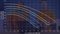In the following guide you will learn what is turnover rate of your pump and how to calculate it.

Turnover rate is the number of hours it takes for the total volume of the pool to pass through the filtration. According to the Gage-Bidwell Law of Dilution, water that is recirculated three to four times per day, or every six to eight hours, will achieve 95 to 98 percent dilution of contaminated pool water with water that has been filtered and disinfected.

This essentially means that for your pool water to be adequately disinfected and filtered, the entire volume of water needs to pass through the filter and sanitation system at least three to four times per day. In order to do this, you will need to set your pool pump at the correct flow rate for the size of your pool.

How to calculate?

To achieve the required Turnover Rate (TR), there must be sufficient flow of water or Flow Rate (FR)

Formula for Turnover Rate:
Divide the pool shape into areas of constant depth, or constant slopes.
Turnover Rate(TR) = Gallons divided by Flow Rate (FR) divided by 60.
Flow Rate (FR) is measured by a flow meter.

Example:
You have a 65,000 gallon pool, and the flow meter is reading 240 GPM (gallons per minute). What is the Turnover Rate (TR)?

Turnover Rate(TR) = Gallons divided by Flow Rate (FR) divided by 60 Gallons = 65,000; FR = 240 GPM

TR = 65,000 gallons ÷ 240 GPM ÷ 60 = 4.5 hours

The standard for a swimming pool is 6 hours. This pool is well within the code standards. Remember, Always operate in compliance with your local or state code requirements.

If you have any other questions about pool and spa products please do let us know - we are here to help!